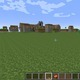Unresolved Detail In Plotted Equations

Sometimes the calculator detects that an equation is too complicated to plot perfectly in a reasonable amount of time. When this happens, the equation is plotted at lower resolution.

216 out of 241 found this helpful
Have more questions? Submit a request

•Jhuber
•CalculusMaster

This one is quite crazy:

\sin \left(10x^2\right)=\cos \left(10y^2\right)

Placing this in the 3-d calculator is also pretty cool

\sin \left(10x^2\right)\cos \left(10y^2\right)

Edited by CalculusMaster
•Harsimar Singh

floor(x)+floor(y)=4

•Jhuber

for a hexagon generater, press playhttps://www.desmos.com/calculator/3io8d6l4ey

•Jhuber
•Mikael Juutinen

y=\log _x\left(x^y\right) no just no

•Ethan LI

check this out, it is so wierd like wth???

https://www.desmos.com/calculator/xp9tttgfzd

•Koulatko

craziest equation in the universe:

\frac{\frac{\cos \left(\tan \left(\sec \left(\frac{x}{y^x}\right)^{\tan \left(\cos \left(x\right)^y\right)}\right)\cdot \sec \left(\frac{x}{y^5}\right)\right)}{\frac{\arctan \left(\cos \left(xy\right)^{\left(\tan \left(\cos \left(x\cdot \sec \left(y\right)\right)\right)\right)}\right)\cdot \sec \left(\tan \left(x^{y^x}\right)\right)}{\frac{\tan \left(\sec \left(x^{y^{\cos \left(xy^{x^y}\right)}}\right)\right)}{\frac{\sec \left(xy\right)}{\sin \left(\tan \left(\cos \left(\sec \left(xy^{4^{\cos \left(xy\right)}}\right)\right)\right)\right)}}}}\cdot \tan \left(\cos \left(\pi \cdot \frac{\cos \left(\frac{xy}{\tan \left(xy\right)}\right)}{\sec \left(\frac{\sin \left(x\right)}{\arctan \left(\cos \left(\arcsin \left(\tan \left(x^{y^{x^y}}\right)\right)\right)\right)}\right)}\right)\right)}{\arcsin \left(\cos \left(\tan \left(x^{\frac{y}{x}}\right)^{\arctan \left(\cos \left(\sin \left(\tan \left(xy\right)\right)\right)\right)}\right)\right)}=\cos \left(\sec \left(xy\right)\right)

•Michal Nemecek

(X^a)+(y^a)=1

a=1000

•Matthew Melgoza

x=cos(y)/tan(x)

•Monkey D Luffy

x!=y

•Michael Millo

Sometimes, if you zoom in enough, the calculator can print finer lines, and the error won't appear.

Zoomed in close enough, x^y=y^x is viewed perfectly, but zoomed out, it cannot calculate perfectly every intricate line in that space.

•Tyler “TySkyo” Skywalker

wy=zx, if w=1/y and z=1/x

•Indevrus

tg(x)+ctg(x)=siny

•Pomona CH

\cos y^x=\tan \ x^y

•Urav Maniar

Plot y=\ \ln e^x

Zoom till range: -2 E-15 <= x <= 2 E-15

-1 E-15 <= y <= 1 E-15.

Edited by Urav Maniar
•H4x0r Jackson (H4x0r)

Looked like some sort of language!

to me anyway

x = nCr(x,y)

Same goes with nPr

•CalculusMaster

\sin \left(xy^2\right)=x

Interesting graph...

•CalculusMaster

\left(\operatorname{floor}\left(x\right)\right)^{\pi }y^{2\pi }=y

•Urav Maniar
•Jerry Lee

MATH
A stupid person typed the following to Desmos:
y=xy(x+y)(x-y)(2x+y)(2x-y)(x+2y)(x-2y)(x^2+y^2-1)(x^2-1)(x^2+y^2-4)(y^2-4)......(x^2+y^2-n^2)(x^2 or y^2 -n^2)
and got this message.
What is the minimum of n?

•Jerry Lee

tan(x)+tan(y)=cos(xy)

Interesting.

•Evan Bailey

The inequality e^x>x+1+0y (the +0y to prevent Desmos from seeing it as a complex inequality on just x, which it cannot graph) behaves strangely at very close zooms and pops an unresolved detail error. To force it to work at larger zooms, copy and paste the following, changing both of the 9's to reflect the desired scale. e^{\frac{x}{10^9}}>1+\frac{x}{10^9}+0y

(you can also set n to a slider starting at 9 and copy and paste this in: e^{\frac{x}{10^n}}>1+\frac{x}{10^n}+0y)

•Albert Zeller

Try sin(y^x*x^y)=cos(y^x*x^y)

•Alajbegua

\cot \left(x^2+y^2\right)=y

•Nate East

\left(\frac{x^{69}}{1^{69}}-\frac{y^{69}}{1^{69}}\right)^2=1

this just makes a square (kinda) that has very high detail corners

•Robert Clarahan

x=\ln \left(yx\sin \left(xy\right)\right)

which is ln( y*x*sin(x*y))

really screws it up.

Is there a way to tell it to take the time to graph it finely?

•Maxim

\left(x^2+y^2-1\right)^3-x^2y^3=0

•Tpierson0958

\sin \left(\frac{\left|y\right|}{-y+\operatorname{sign}\left(y\right)}\right)=\cos \left(\frac{\left|x\right|}{-x+\operatorname{sign}\left(x\right)}\right)

•Tpierson0958

\sin \left(22\left(\frac{\left|\sqrt{y^2+x^2}\right|}{\operatorname{sign}\left(\sqrt{y^2+x^2}\right)-\sqrt{y^2+x^2}}\cdot \sin \left(\arctan \left(\frac{y}{x}\right)\right)\right)\right)=\sin \left(22\left(\left(\frac{\left|\sqrt{y^2+x^2}\right|}{\operatorname{sign}\left(\sqrt{y^2+x^2}\right)-\sqrt{y^2+x^2}}\right)\cos \left(\arctan \left(\frac{y}{x}\right)\right)\right)\right)